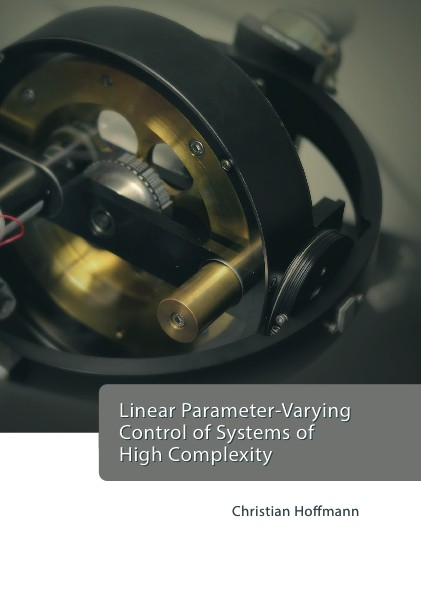Datenbestand vom 25. Mai 2023aktualisiert am 25. Mai 2023

# ISBN 978384392682996,00 € inkl. MwSt, zzgl. Versand

Christian Hoffmann
Linear Parameter-Varying Control of Systems of High Complexity

401 Seiten, Dissertation Technische Universität Hamburg-Harburg (2015), Hardcover, A5

## Zusammenfassung / Abstract

The present work considers plant representations in the framework of linear parameter-varying (LPV) systems that may involve a high degree of complexity. This class contains nonlinear systems that lead to high costs in modeling, synthesis and implementation of associated LPV controllers on the one hand as well as systems consisting of a large number of LPV subsystems interconnected through a possibly time-varying topology on the other hand. For complex LPV systems of the first kind, the contribution of this thesis consists in the development of systematic tools for mathematically exact modeling with reduced complexity and the subsequent efficient exploitation by extended LPV synthesis methods. The fundamental approach follows a translation of nonlinear differential equations into a descriptor state space LPV representation, parameterization and possible approximation by means of a principle component analysis. The synthesis conditions and implementation are analyzed in terms of their respective computational effort and reduced by mathematically equivalent modification. The methods are validated on nonlinear models of an industrial robot and a Control Moment Gyroscope (CMG). LPV controllers are synthesized for both the exact as well as the approximated models and experimentally implemented with low computational costs. Furthermore, a general framework for the representation of distributed LPV systems is introduced. A straightforward formulation of synthesis conditions for the entire system allows reducing the synthesis complexity via the introduction of structural constraints on decision variables. In this vein, synthesis conditions are formulated whose complexity ranges in the order of a single subsystem times the number of different subsystem dynamics. The diagonalizability of interaction matrices is achieved by a transformation that leads to virtual symmetric or normal interaction matrices. Such matrices can be diagonalized by unitary transformations, which allows the direct congruence transformation of synthesis conditions for the consideration of time-varying, directed topologies. The presented methods are evaluated against state-of-the-art techniques and validated in a numerical example as well as in a simulated leader-follower-based formation of a group of heterogeneous nonlinear quadrotor helicopters interconnected through arbitrary directed topologies.